# Information on specular, SOV and Complete SOV models

During the simulation of an echo, applying the principle of reciprocity consists in calculating for each point of the defect:

• the emission beam,
• the receiving beam
• and a diffraction coefficient adapted to the control mode.

In the current implementation, CIVA considers the beams in transmission and reception as planar wavefronts from which will be extracted a time of flight, an amplitude, a phase, a propagation direction and a polarization (method calles "beam max extraction). However, when the defect is located in the near field or when the beam arriving on the default is composed of several contributions (eg due to a reflection on different part of the specimen) this approximation is no longer valid. Similarly, when dealing with phased-array simulation, the effects associated with the presence of grating lobes are not considered.

The principle of the "COMPLETE_SOV" model  and "COMPLETE_KIRCHHOFF" model is to overcome this limitation by not simplifying the description of the beam but by exploiting directly from the rays coming from the probes in transmission and reception.

## The SOV model

The Interaction "beam/spherical inclusion" is calculated by assuming a plane wave incident on the spherical inclusion (Figure 1). This  "plane wave" approximation consists in describing the incident field on the spherical inclusion with a time of flight, an amplitude, a phase, a propagation direction and polarization (method called "max beam extraction").

These characteristics of the field are calculated at the center of the inclusion and forwarded to the SOV model that calculates the 3D echo of inclusion.

The phase of the sphere echo, calculated using 3D diffraction coefficients takes into account the time of flight offset between the shining point of the inclusion and its center (Figure 1). The resulting echo is correctly positioned at the shinning point of the sphere.

The SOV model is an exact model that includes:

•  the specular echo of the sphere
• the contribution of the creeping wave
• and waves penetrating and propagating in the sphere.

Note: What is valid for the incident field is also valid for the received beam because of the reciprocity principle used to calculate the echoes with the SOV model.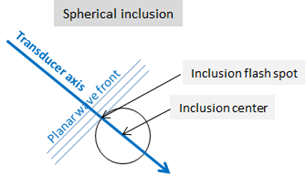The SOV model is not valid for large ka, due to numerical problems in the calculation of coefficients.

The approximation "plane wave" is not valid when the sphere is large.

For example, Figure 2 illustrates the limit of validity of the approximation "plane wave" in the case of a sphere of large radius "a" with respect to the dimension of the focal spot of the incident field.

It is seen that the amplitude of the incident field varies on the surface contributing to the echo of the sphere (called "specular area" and of arbitrary size in the figure). However, these variations are not taken into account because the SOV model assumes that the amplitude of the incident plane wave is the one measured at the center of the sphere.

This approximation of the amplitude of the incident field is valid as long as the specular area of the sphere (related to its radius, the size and position of the probe relative to the sphere) is not too large compared to the beam .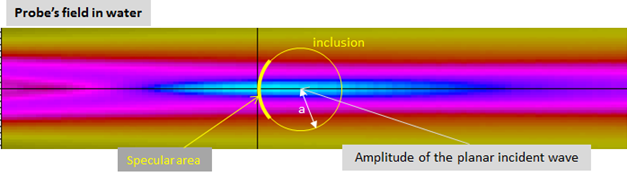The approximation of using the incident field in the center of the sphere is not valid when the sphere is large. Even if the incident field varies little over the surface that contributes to the echo of the sphere, using the amplitude and direction of the calculated incident field at the center of the sphere while the echo is generated by the specular area around the bright spot, can induce an error. This approximation is valid as long as the incident fields at the bright spot in the center of the sphere are not too different, that is to say as long as the radius "a" of the sphere is not too large compared to the changes in the field between the bright spot and the center (Figure 3).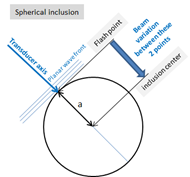This validity limit of the planar wave approximation related to the size of the sphere with respect to the spot or the focal length of the beam has been illustrated by considering the amplitude of the beam because it is easy to view. However it concerns also the propagation direction, the time of flight and phase of the incident field.

The approximation "plane wave" is not valid when the sphere is located in the near field or when the field spread on the defect imply several contributions, eg due to a reflection on different part of the specimen.

These limitations are due to the taking into accoiunt of a single time of flight to describe the incident field.

These examples of the limits the SOV model show that it is difficult to find a simple criterion to define the domain of validity of the model.

The bridles exist in CIVA to translate the limitations related to the size of the sphere with respect to characteristic values of the incident field. These bridles are based on the value of the ratio of the radius of the sphere and the wavelength of the incident waves as it is linked to the characteristics of the field. This value must not exceed a threshold defined empirically.

The limits of the SOV model related to the consideration of a single time of flight are not translated by bridles in CIVA.

## The complete_sov model

With the SOV model, while computing the echo of the sphere, the global incident field (that is to say the field formed by all rays from the probe) on inclusion is approached by a planar wave.

With the COMPLETE_SOV model, the field associated with each ray from an elementary surface of the probe is approached by a plane wave (Figure 4). Juste like SOV, each plane wave is described by a time of flight, an amplitude, a phase, a propagation direction and polarization. These characteristics are calculated at the center of the inclusion and then transmitted to the SOV model which calculates the 3D echo of the inclusion for each ray. Then the echoes obtained for each ray are summed to obtain the final echo of the inclusion.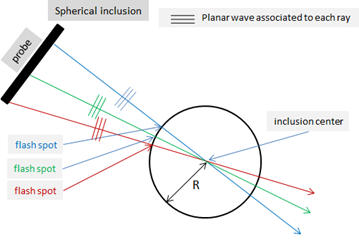Similar considerations to those of the SOV model determine the limits of validity of COMPLETE_SOV model. However, the fact of considering as many plane waves as rays causes less limitations.

For example, the field associated with a ray is not focused and does not vary much on the specular surface of the sphere. The beam variation of one single ray between the bright spot and the center of the sphere is only due to divergence and varies less than in the case of a global beam from a focused probe. One time of flight is sufficient to describe it; the problem of the extraction of a single time of flight that does not allow to properly describe the global near field of a probe no longer exists.

On the other hand, the limits due to the spectral overlap are the same as for SOV.

## Comparison SOV vs Complete_SOV

According to the descriptions of these two models, the COMPLETE_SOV model should better simulate echoes of sphere than SOV when they are :

• located in the near field of the probe
• insonified by multiple contributions
• of larger diameter than the SOV model considering the limitation related to the description of the field on the surface of the sphere. On the other hand, the diffraction limit associated with coefficients which are false for large 'ka' is the same as for SOV.

In the far field, the predictions of the two models are close because the rays coming from the probe and taken into account in the COMPLETE_SOV model are parallel to the surface of the spheres, in the direction of the plane wave associated with the field of the probe.

## The SPECULAR model

Only the specular echo is simulated. It takes into account only the rays coming from the probe, reflected on the surface of the sphere and going back to to the probe. The reflection coefficients of these rays on the surface of the sphere are "3D" and depend on the medium in the sphere.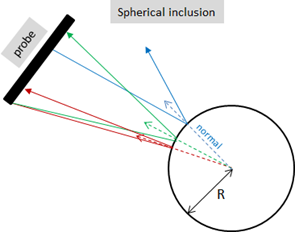The advantages of this model (which considers as many elementary fields as there are rays coming from the probe) are the same as for the COMPLETE_SOV model. Moreover, the beam used as input data in the SPECULAR model is calculated at the intersection point of each ray with the surface of the sphere where the echo is formed rather than in its center.

However, this model does not take into account the diffraction phenomena. It is therefore valid for the large spheres with respect to the wavelength.

It calculates nor creeping waves, or waves penetrating the inclusion.